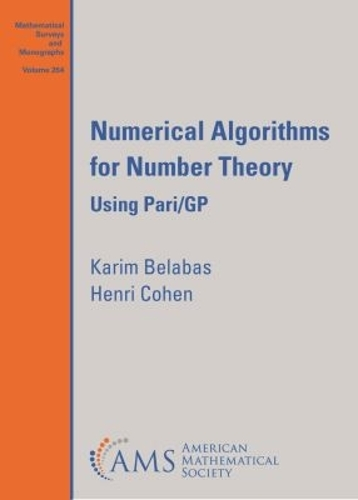•# Numerical Algorithms for Number Theory: Using Pari/GP - Mathematical Surveys and Monographs (Paperback)

(author), (author)
£103.00
Paperback 430 Pages / Published: 30/08/2021
• We can order this from the publisher

Usually dispatched within 10 working days

This book presents multiprecision algorithms used in number theory and elsewhere, such as extrapolation, numerical integration, numerical summation (including multiple zeta values and the Riemann-Siegel formula), evaluation and speed of convergence of continued fractions, Euler products and Euler sums, inverse Mellin transforms, and complex \$L\$-functions. For each task, many algorithms are presented, such as Gaussian and doubly-exponential integration, Euler-MacLaurin, Abel-Plana, Lagrange, and Monien summation. Each algorithm is given in detail, together with a complete implementation in the free Pari/GP system. These implementations serve both to make even more precise the inner workings of the algorithms, and to gently introduce advanced features of the Pari/GP language. This book will be appreciated by anyone interested in number theory, specifically in practical implementations, computer experiments and numerical algorithms that can be scaled to produce thousands of digits of accuracy.

Publisher: American Mathematical Society
ISBN: 9781470463519
Number of pages: 430
Dimensions: 254 x 178 mm